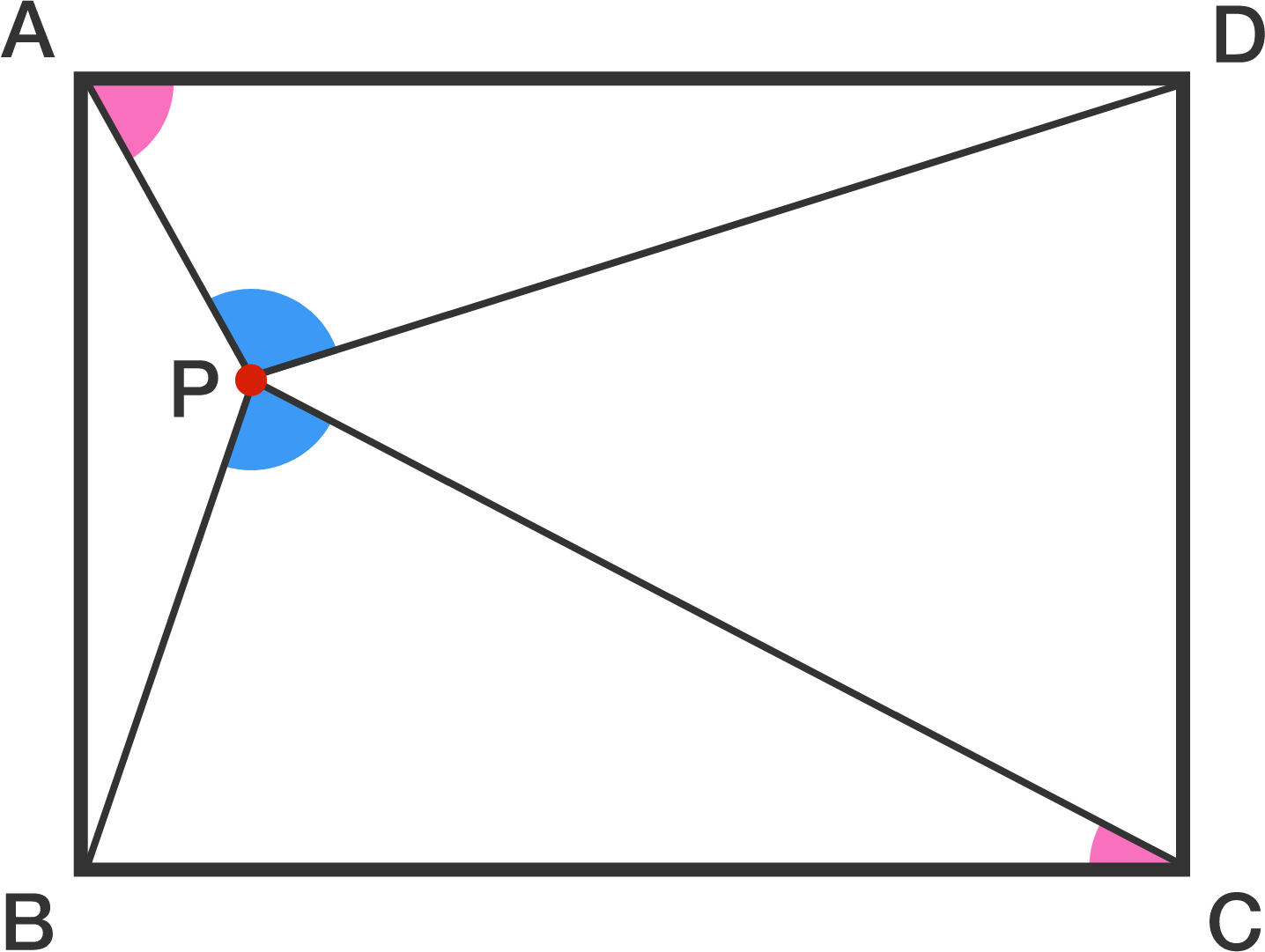# Too Many Angles But Insufficient Information!

Geometry Level 2An interior point $P$ is chosen in the rectangle $ABCD$ such that the sum of angles $APD$ and $BPC$ is $180^\circ$. Find the sum of angles $\angle DAP$ and $\angle BCP$ in degrees.

×